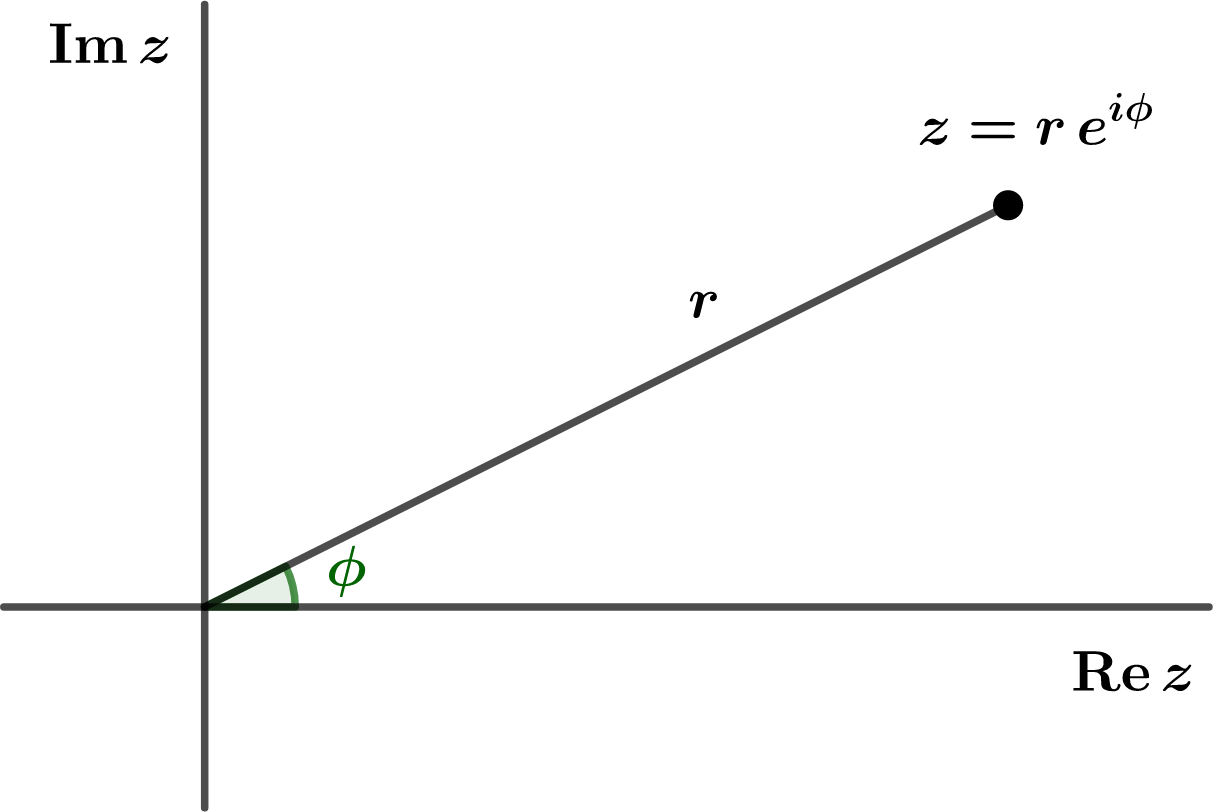## Section2.6Exponential Form

If we use polar coordinates

\begin{align} x\amp = r\cos\theta\tag{2.6.1}\\ y\amp = r\sin\theta\tag{2.6.2} \end{align}

to describe the complex number $$z=x+iy\text{,}$$ we can factor out the $$r$$ and use Euler's formula to obtain the exponential form of the complex number $$z\text{:}$$

\begin{align} z\amp = x+iy\notag\\ \amp = r\cos\theta + i r\sin\theta\notag\\ \amp = r(\cos\theta +i\sin\theta)\notag\\ \amp = re^{i\theta}\tag{2.6.3} \end{align}

Just as $$r$$ represents the distance of $$z$$ from the origin in the complex plane, $$\theta$$ represents the polar angle, measured in radians, counterclockwise from the real axis, as shown in Figure 2.6.1Figure 2.6.1. The point $$z=re^{i\theta}\text{.}$$

It is easiest to do multiplication and division of two complex numbers $$z_1=r_1e^{i\theta_1}$$ and $$z_2 = r_2 e^{i\theta_2}$$ in exponential form:

\begin{align} z_1 z_2 \amp = r_1 e^{i\theta_1}\, r_2 e^{i\theta_2}\notag\\ \amp = r_1 r_2 e^{i(\theta_1 + \theta_2)}\tag{2.6.4}\\ \frac{z_1}{ z_2} \amp = r_1 e^{i\theta_1}/ r_2 e^{i\theta_2}\notag\\ \amp = \frac{r_1}{ r_2} e^{i(\theta_1 - \theta_2)}\tag{2.6.5} \end{align}

Notice that the magnitudes of the two complex numbers multiply (or divide) whereas the angles add (or substract).